# On symmetric positive homoclinic solutions of semilinear p-Laplacian differential equations

## Abstract

In this paper we study the existence of even positive homoclinic solutions for p-Laplacian ordinary differential equations (ODEs) of the type ${\left({u}^{\mathrm{\prime }}{|{u}^{\mathrm{\prime }}|}^{p-2}\right)}^{\mathrm{\prime }}-a\left(x\right)u{|u|}^{p-2}+\lambda b\left(x\right)u{|u|}^{q-2}=0$, where $2\le p, $\lambda >0$ and the functions a and b are strictly positive and even. First, we prove a result on symmetry of positive solutions of p-Laplacian ODEs. Then, using the mountain-pass theorem, we prove the existence of symmetric positive homoclinic solutions of the considered equations. Some examples and additional comments are given.

MSC:34B18, 34B40, 49J40.

## 1 Introduction and main results

In this paper we prove the existence of positive homoclinic solutions for p-Laplacian ODEs of the type

${\left({u}^{\mathrm{\prime }}{|{u}^{\mathrm{\prime }}|}^{p-2}\right)}^{\mathrm{\prime }}-a\left(x\right)u{|u|}^{p-2}+\lambda b\left(x\right)u{|u|}^{q-2}=0,\phantom{\rule{1em}{0ex}}x\in \mathbb{R},$
(1)

where $2\le p and $\lambda >0$. We assume that

(H) the functions $a\left(x\right)$ are $b\left(x\right)$ are continuously differentiable, strictly positive, $0 and $0. Let, moreover, $a\left(x\right)$ and $b\left(x\right)$ be even functions on , $x{a}^{\mathrm{\prime }}\left(x\right)>0$ and $x{b}^{\mathrm{\prime }}\left(x\right)<0$ for $x\ne 0$.

By a solution of (1), we mean a function $u:\mathbb{R}\to \mathbb{R}$ such that $u\in {C}^{1}\left(\mathbb{R}\right)$, ${\left({u}^{\mathrm{\prime }}{|{u}^{\mathrm{\prime }}|}^{p-2}\right)}^{\mathrm{\prime }}\in C\left(\mathbb{R}\right)$ and Eq. (1) holds for every $x\in \mathbb{R}$. We are looking for positive solutions of (1) which are homoclinic, i.e., $u\left(x\right)\to 0$ and ${u}^{\mathrm{\prime }}\left(x\right)\to 0$ as $|x|\to \mathrm{\infty }$.

In the case $p=2$, $q=4$ and $\lambda =1$, similar problems are considered in  using variational methods. Note that in  and  the following second-order differential equations are considered:

${u}^{\mathrm{\prime }\mathrm{\prime }}-a\left(x\right)u-b\left(x\right){u}^{2}+c\left(x\right){u}^{3}=0$

and

${u}^{\mathrm{\prime }\mathrm{\prime }}+a\left(x\right)u-b\left(x\right){u}^{2}+c\left(x\right){u}^{3}=0,$

where a, b and c are periodic, bounded functions and a and c are positive. These equations come from a biomathematics model suggested by Austin  and Cronin . Further results and the phase plane analysis of these equations with constant coefficients are given in . Note that the periodic and homoclinic solutions of p-Laplacian ODEs are considered in [7, 8].

The present work is an extension of these studies to p-Laplacian ODEs. Let ${X}_{T}:={W}_{0}^{1,p}\left(-T,T\right)$ be the Sobolev space of p-integrable absolutely continuous functions $u:\left[-T,T\right]\to \mathbb{R}$ such that

${\parallel u\parallel }^{p}={\int }_{-T}^{T}\left({|{u}^{\mathrm{\prime }}\left(x\right)|}^{p}+{|u\left(x\right)|}^{p}\right)\phantom{\rule{0.2em}{0ex}}dx<\mathrm{\infty }$

and $u\left(-T\right)=u\left(T\right)=0$.

We use a variational treatment of the problem considering the functional ${J}_{T}:{X}_{T}\to \mathbb{R}$

${J}_{T}\left(u\right)={\int }_{-T}^{T}\left(\frac{1}{p}\left({|{u}^{\mathrm{\prime }}\left(x\right)|}^{p}+a\left(x\right){|u\left(x\right)|}^{p}\right)-\frac{\lambda }{q}b\left(x\right){\left({u}^{+}\left(x\right)\right)}^{q}\right)\phantom{\rule{0.2em}{0ex}}dx,$

where ${u}^{+}\left(x\right)=max\left\{u\left(x\right),0\right\}$.

Using the well-known mountain-pass theorem, we conclude that the functional ${J}_{T}$ has a nontrivial critical point ${u}_{T,\lambda }\in {X}_{T}$, which is a solution of the restricted problem

$\begin{array}{r}{\left({u}^{\mathrm{\prime }}{|{u}^{\mathrm{\prime }}|}^{p-2}\right)}^{\mathrm{\prime }}-a\left(x\right)u{|u|}^{p-2}+\lambda b\left(x\right)u{|u|}^{q-2}=0,\phantom{\rule{1em}{0ex}}x\in \left(-T,T\right),\\ u\left(-T\right)=u\left(T\right)=0.\end{array}$
(2)

Further, we obtain uniform estimates for the solutions ${u}_{T,\lambda }$, extended by 0 outside $\left[-T,T\right]$. Then, a positive homoclinic solution ${u}_{\lambda }$ of (1) is found as a limit of ${u}_{T,\lambda }$, as $T\to \mathrm{\infty }$ in ${C}_{\mathrm{loc}}^{1}\left(\mathbb{R}\right)$. The function ${u}_{\lambda }$ is also an even function.

To obtain the property, we extend the symmetry lemma of Korman and Ouyang  to the p-Laplacian equations. The result is formulated and proved in Section 2.

Our main result is:

Theorem 1 Suppose that $2\le p, $\lambda >0$ and assumptions (H) hold. Then Eq. (1) has a positive solution ${u}_{\lambda }$ such that ${u}_{\lambda }\left(x\right)\to 0$ and ${u}_{\lambda }^{\mathrm{\prime }}\left(x\right)\to 0$ as $|x|\to \mathrm{\infty }$. Moreover, the solution ${u}_{\lambda }$ is an even function, $max\left\{{u}_{\lambda }\left(x\right):x\in \mathbb{R}\right\}={u}_{\lambda }\left(0\right)\to +\mathrm{\infty }$ as $\lambda \to 0$ and ${u}_{\lambda }^{\mathrm{\prime }}\left(x\right)<0$ for $x>0$.

Theorem 1 is proved in Section 3. From its proof we have

$max\left\{{u}_{\lambda }\left(x\right):x\in \mathbb{R}\right\}={u}_{\lambda }\left(0\right)\ge {\left(\frac{a\left(0\right)}{\lambda b\left(0\right)}\right)}^{1/\left(q-p\right)}>0,$

from which it follows that ${u}_{\lambda }\left(0\right)\to +\mathrm{\infty }$ as $\lambda \to 0$. Observe that if $\lambda =0$, the problem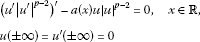has a unique solution $u=0$. Indeed, multiplying the equation by u and integrating by parts over , we obtain

${\int }_{-\mathrm{\infty }}^{\mathrm{\infty }}\left({|{u}^{\mathrm{\prime }}\left(x\right)|}^{p}+a\left(x\right){|u\left(x\right)|}^{p}\right)\phantom{\rule{0.2em}{0ex}}dx=0,$

which implies that $u\equiv 0$.

A simplified method can be applied to the equations

${u}^{\mathrm{\prime }\mathrm{\prime }}-a\left(x\right)u{|u|}^{p-2}+\lambda b\left(x\right)u{|u|}^{q-2}=0,\phantom{\rule{1em}{0ex}}x\in \mathbb{R},$
(3)

under assumptions (H) and $2\le p, $\lambda >0$. Note that in this case, the even homoclinic solution ${u}_{\lambda }$ of Eq. (3) satisfies

$max\left\{{u}_{\lambda }\left(x\right):x\in \mathbb{R}\right\}={u}_{\lambda }\left(0\right)\ge {\left(\frac{a\left(0\right)}{\lambda b\left(0\right)}\right)}^{1/\left(q-p\right)},$

and again ${u}_{\lambda }\left(0\right)\to +\mathrm{\infty }$ as $\lambda \to 0$. If a and b are constants, Eq. (3) is a conservative system and one can plot the phase curves ${\left(\frac{v}{2}\right)}^{2}-a\frac{{|u|}^{p}}{p}+\lambda b\frac{{|u|}^{q}}{q}=C$ in the phase plane $\left(u,v\right)=\left(u,{u}^{\mathrm{\prime }}\right)$. An example is given at the end of Section 3.

## 2 Preliminary results

Let ${\phi }_{p}\left(t\right)=t{|t|}^{p-2}$, $p\ge 2$ and ${\mathrm{\Phi }}_{p}\left(t\right)=\frac{{|t|}^{p}}{p}$. It is clear that ${\mathrm{\Phi }}_{p}\left(t\right)$ is a differentiable function and ${\mathrm{\Phi }}_{p}^{\mathrm{\prime }}\left(t\right)={\phi }_{p}\left(t\right)$. Moreover, ${\phi }_{p}^{\mathrm{\prime }}\left(t\right)$ exists and ${\phi }_{p}^{\mathrm{\prime }}\left(t\right)=\left(p-1\right){|t|}^{p-2}$ for $p\ge 2$.

Let ${L}^{p}\left(a,b\right)$, $1 be the space of Lebesgue measurable functions $u:\left(a,b\right)\to \mathbb{R}$ such that the norm ${|u|}_{p}^{p}={\int }_{a}^{b}{|u\left(x\right)|}^{p}\phantom{\rule{0.2em}{0ex}}dx<\mathrm{\infty }$.

The dual space of ${L}^{p}\left(a,b\right)$ is ${L}^{{p}^{\mathrm{\prime }}}\left(a,b\right)$, where $\frac{1}{p}+\frac{1}{{p}^{\mathrm{\prime }}}=1$. Let $〈\cdot ,\cdot 〉$ be the duality pairing between ${L}^{{p}^{\mathrm{\prime }}}\left(a,b\right)$ and ${L}^{p}\left(a,b\right)$. By the Hölder inequality, $|〈v,u〉|\le {|v|}_{{p}^{\mathrm{\prime }}}{|u|}_{p}$ for any $v\in {L}^{{p}^{\mathrm{\prime }}}\left(a,b\right)$ and $u\in {L}^{p}\left(a,b\right)$. We will use the following lemmata in further considerations.

Lemma 2 For any $u,v\in {L}^{p}\left(a,b\right)$, the following inequality holds:

$〈{\phi }_{p}\left(u\right)-{\phi }_{p}\left(v\right),u-v〉\ge \left({|u|}_{p}^{p-1}-{|v|}_{p}^{p-1}\right)\left({|u|}_{p}-{|v|}_{p}\right).$

Proof of Lemma 2. Note that for $u\in {L}^{p}\left(a,b\right)$, ${\phi }_{p}\left(u\right)\in {L}^{{p}^{\mathrm{\prime }}}\left(a,b\right)$. From the Hölder inequality, we have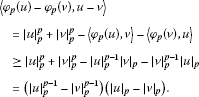□

Lemma 3 Let $p\ge 2$, $u\in {C}^{1}\left(\left[a,b\right]\right)$ and ${\left({u}^{\mathrm{\prime }}{|{u}^{\mathrm{\prime }}|}^{p-2}\right)}^{\mathrm{\prime }}\in C\left(\left[a,b\right]\right)$. Then

${\int }_{a}^{b}{\left({u}^{\mathrm{\prime }}{|{u}^{\mathrm{\prime }}|}^{p-2}\right)}^{\mathrm{\prime }}{u}^{\mathrm{\prime }}\phantom{\rule{0.2em}{0ex}}dx=\frac{p-1}{p}\left({|{u}^{\mathrm{\prime }}\left(b\right)|}^{p}-{|{u}^{\mathrm{\prime }}\left(a\right)|}^{p}\right).$

The statement of Lemma 3 follows simply from the identity

${\left({|{u}^{\mathrm{\prime }}|}^{p}\right)}^{\mathrm{\prime }}=\frac{p}{p-1}{\left({u}^{\mathrm{\prime }}{|{u}^{\mathrm{\prime }}|}^{p-2}\right)}^{\mathrm{\prime }}{u}^{\mathrm{\prime }}.$

The one-dimensional p-Laplacian operator ${L}_{p}$ for a differentiable function u on the interval I is introduced as ${L}_{p}\left(u\right):={\left({\phi }_{p}\left({u}^{\mathrm{\prime }}\right)\right)}^{\mathrm{\prime }}$. Let us consider the problem

$\left\{\begin{array}{c}{L}_{p}\left(u\right)+f\left(x,u\right)=0,\phantom{\rule{1em}{0ex}}x\in \left(-T,T\right),\hfill \\ u\left(-T\right)=u\left(T\right)=0,\hfill \end{array}$
(4)

where $f\in {C}^{1}\left(\left[-T,T\right]×{\mathbb{R}}^{+}\right)$ and satisfies

$\begin{array}{r}f\left(-x,u\right)=f\left(x,u\right),\phantom{\rule{1em}{0ex}}x\in \left(-T,T\right),u>0,\\ x{f}_{x}\left(x,u\right)<0,\phantom{\rule{1em}{0ex}}x\in \left(-T,T\right)\mathrm{\setminus }\left\{0\right\},u>0.\end{array}$
(5)

A function $u:\left[-T,T\right]\to \mathbb{R}$ is said to be a solution of the problem (4) if $u\in {C}^{1}\left(\left[-T,T\right]\right)$ with $u\left(-T\right)=u\left(T\right)=0$ is such that ${u}^{\mathrm{\prime }}{|{u}^{\mathrm{\prime }}|}^{p-2}$ is absolutely continuous and ${L}_{p}u\left(x\right)+f\left(x,u\left(x\right)\right)=0$ holds a.e. in $\left(-T,T\right)$.

We formulate an extension of Lemma 1 of  for p-Laplacian nonlinear equations. The result of Korman and Ouyang is one-dimensional analogue of the result of Gidas, Ni and Nirenberg  for symmetry of positive solutions of semilinear Laplace equations. In the case of p-Laplacian equations, the symmetry of solutions in higher dimensions is discussed by Reihel and Walter .

Theorem 4 Assume that $f\in {C}^{1}\left(\left[-T,T\right]×{\mathbb{R}}^{+}\right)$ satisfies (5). Then any positive solution u of (4) is an even function such that $max\left\{u\left(x\right):-T\le x\le T\right\}=u\left(0\right)$ and ${u}^{\mathrm{\prime }}\left(x\right)<0$ for $x\in \left(0,T\right]$.

Remark 1 Let us note that if the function f satisfies (5), but u is not a positive solution of (4), then u is not necessarily an even function. A simple counter example in the case $p=2$ is the problem

$\left\{\begin{array}{c}{u}^{\mathrm{\prime }\mathrm{\prime }}+u-{x}^{2}+{\pi }^{2}-2=0,\phantom{\rule{1em}{0ex}}-\pi

The term $f\left(x,u\right)=u-{x}^{2}+{\pi }^{2}-2$ satisfies (5) in the interval $\left(-\pi ,\pi \right)$, but the solution of the problem $u\left(x\right)={x}^{2}-{\pi }^{2}+sinx$ is negative in $\left(-\pi ,\pi \right)$ and not an even function. Its graph is presented in Figure 1. It would be more interesting to show an example for the case $p>2$ and f satisfying the additional assumption $f\left(x,0\right)=0$.

Sketch of Proof of Theorem 4 Suppose that the function u has only one global maximum on $\left[-T,T\right]$.

Assume that the function $u\left(x\right)$ has a finite number of local minima in the interval $\left[0,T\right]$, and let ${x}_{1}$ be the largest local minimum. Let $\overline{x}\in \left[{x}_{1},T\right]$ be the local maximum and $\stackrel{˜}{x}\in \left[\overline{x},T\right]$ be such that $u\left({x}_{1}\right)=u\left(\stackrel{˜}{x}\right)$. Denote ${u}_{1}=u\left({x}_{1}\right)=u\left(\stackrel{˜}{x}\right)$ and ${u}_{2}=u\left(\overline{x}\right)$, and let $x=\alpha \left(u\right)$ and $x=\beta \left(u\right)$ be the inverse functions of the function $u=u\left(x\right)$ in the intervals $\left[{x}_{1},\overline{x}\right]$ and $\left[\overline{x},T\right]$, respectively. Multiplying the equation in (4) by ${u}^{\mathrm{\prime }}$ and integrating in $\left[{x}_{1},\stackrel{˜}{x}\right]$, we obtain by Lemma 3 and (5):

$\begin{array}{rcl}0& =& {\int }_{{x}_{1}}^{\stackrel{˜}{x}}\left({L}_{p}\left(u\right){u}^{\mathrm{\prime }}+f\left(x,u\right){u}^{\mathrm{\prime }}\right)\phantom{\rule{0.2em}{0ex}}dx\\ =& \frac{p-1}{p}{|{u}^{\mathrm{\prime }}|}^{p}\left(\stackrel{˜}{x}\right)+{\int }_{{x}_{1}}^{\overline{x}}f\left(x,u\right){u}^{\mathrm{\prime }}\phantom{\rule{0.2em}{0ex}}dx+{\int }_{\overline{x}}^{\stackrel{˜}{x}}f\left(x,u\right){u}^{\mathrm{\prime }}\phantom{\rule{0.2em}{0ex}}dx\\ =& \frac{p-1}{p}{|{u}^{\mathrm{\prime }}|}^{p}\left(\stackrel{˜}{x}\right)+{\int }_{{u}_{1}}^{{u}_{2}}\left(f\left(\alpha \left(u\right),u\right)-f\left(\beta \left(u\right),u\right)\right)\phantom{\rule{0.2em}{0ex}}du\\ >& 0,\end{array}$

which leads to contradiction. One can prove the last fact using other arguments; see, for instance, Theorem 2.1 of . Suppose now that u has infinitely many local minima in $\left[-T,{x}^{\ast }\right]$. Further, we can follow the steps of the proof of Lemma 1 of  with corresponding modifications based on Lemma 3. □

## 3 Proof of the main result

Let ${X}_{T}={W}_{0}^{1,p}\left(-T,T\right)$ be the Sobolev space of p-integrable absolutely continuous functions $u:\left[-T,T\right]\to \mathbb{R}$ such that

${\parallel u\parallel }_{T}^{p}={\int }_{-T}^{T}\left({|{u}^{\mathrm{\prime }}\left(x\right)|}^{p}+{|u\left(x\right)|}^{p}\right)\phantom{\rule{0.2em}{0ex}}dx<\mathrm{\infty }$

and $u\left(-T\right)=u\left(T\right)=0$. Note that if $a\left(x\right)$ is strictly positive and bounded, i.e., there exist a and A such that $0, then ${\parallel u\parallel }_{a,T}^{p}={\int }_{-T}^{T}\left({|{u}^{\mathrm{\prime }}\left(x\right)|}^{p}+a\left(x\right){|u\left(x\right)|}^{p}\right)\phantom{\rule{0.2em}{0ex}}dx$ is an equivalent norm in ${X}_{T}$.

We need an extension to the p-case of the following proposition by Rabinowitz .

Proposition 5 Let $u\in {W}_{\mathrm{loc}}^{1,p}\left(\mathbb{R}\right)$. Then:

1. (i)

If $T\ge 1$, for $x\in \left[T-1/2,T+1/2\right]$,

$\underset{x\in \left[T-1/2,T+1/2\right]}{max}|u\left(x\right)|\le {2}^{\frac{p-1}{p}}{\left({\int }_{T-1/2}^{T+1/2}\left({|{u}^{\mathrm{\prime }}\left(t\right)|}^{p}+{|u\left(t\right)|}^{p}\right)\phantom{\rule{0.2em}{0ex}}dt\right)}^{1/p}.$
(6)
2. (ii)

For every $u\in {W}_{0}^{1,p}\left(-T,T\right)$,

${\parallel u\parallel }_{{L}^{\mathrm{\infty }}\left(-T,T\right)}\le {2}^{\frac{p-1}{p}}{\parallel u\parallel }_{T}.$
(7)

Proof of Proposition 5 Let $x,t\in \left[T-1/2,T+1/2\right]$. It follows

$|u\left(x\right)|\le |u\left(t\right)|+{\int }_{T-1/2}^{T+1/2}|{u}^{\mathrm{\prime }}\left(s\right)|\phantom{\rule{0.2em}{0ex}}ds.$

Integrating with respect to $t\in \left[T-1/2,T+1/2\right]$ and using the Hölder and Jensen inequalities, we obtain

$\begin{array}{rcl}|u\left(x\right)|& \le & {\int }_{T-1/2}^{T+1/2}|u\left(t\right)|\phantom{\rule{0.2em}{0ex}}dt+{\int }_{T-1/2}^{T+1/2}|{u}^{\mathrm{\prime }}\left(s\right)|\phantom{\rule{0.2em}{0ex}}ds\\ \le & {\left({\int }_{T-1/2}^{T+1/2}{|u\left(t\right)|}^{p}\phantom{\rule{0.2em}{0ex}}dt\right)}^{1/p}+{\left({\int }_{T-1/2}^{T+1/2}{|{u}^{\mathrm{\prime }}\left(t\right)|}^{p}\phantom{\rule{0.2em}{0ex}}dt\right)}^{1/p}\\ \le & {2}^{\frac{p-1}{p}}{\left({\int }_{T-1/2}^{T+1/2}\left({|{u}^{\mathrm{\prime }}\left(t\right)|}^{p}+{|u\left(t\right)|}^{p}\right)\phantom{\rule{0.2em}{0ex}}dt\right)}^{1/p}.\end{array}$
1. (ii)

Take $u\in {W}_{0}^{1,p}\left(-T,T\right)$. Since ${W}_{0}^{1,p}\left(-T,T\right)\subset C\left[-T,T\right]$, there exists $\tau \in \left[-T,T\right]$ such that by (i)

$\begin{array}{rcl}{\parallel u\parallel }_{{L}^{\mathrm{\infty }}\left(-T,T\right)}& =& {\parallel u\parallel }_{C\left[\tau -1/2,\tau +1/2\right]}\le {2}^{\frac{p-1}{p}}{\left({\int }_{\tau -1/2}^{\tau +1/2}\left({|{u}^{\mathrm{\prime }}\left(t\right)|}^{p}+{|u\left(t\right)|}^{p}\right)\phantom{\rule{0.2em}{0ex}}dt\right)}^{1/p}\\ \le & 2\parallel u\parallel .\end{array}$

□

We are looking for positive solutions of (1), which are homoclinic, i.e., $u\left(x\right)\to 0$ and ${u}^{\mathrm{\prime }}\left(x\right)\to 0$ as $|x|\to \mathrm{\infty }$. Firstly, we look for positive solutions of the problem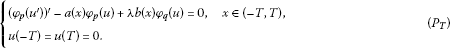A function $u:\left[-T,T\right]\to \mathbb{R}$ is said to be a solution of the problem (${P}_{T}$) if $u\in {C}^{1}\left(\left[-T,T\right]\right)$ with $u\left(-T\right)=u\left(T\right)=0$ is such that ${\phi }_{p}\left({u}^{\mathrm{\prime }}\right)$ is absolutely continuous and ${\left({\phi }_{p}\left({u}^{\mathrm{\prime }}\right)\right)}^{\mathrm{\prime }}\left(x\right)-a\left(x\right){\phi }_{p}\left(u\right)\left(x\right)+\lambda b\left(x\right){\phi }_{q}\left(u\right)\left(x\right)=0$ holds a.e. in $\left(-T,T\right)$.

A function $u:\left[-T,T\right]\to \mathbb{R}$ is said to be a weak solution of the problem (${P}_{T}$) if

${\int }_{-T}^{T}\left({\left({\phi }_{p}\left({u}^{\mathrm{\prime }}\right)\right)}^{\mathrm{\prime }}{v}^{\mathrm{\prime }}\phantom{\rule{0.2em}{0ex}}dx+a\left(x\right){\phi }_{p}\left(u\right)v-\lambda b\left(x\right){\phi }_{q}\left(u\right)v\right)\phantom{\rule{0.2em}{0ex}}dx=0,\phantom{\rule{1em}{0ex}}\mathrm{\forall }v\in {W}_{0}^{1,p}\left(\left(-T,T\right)\right).$

Standard arguments show that a weak solution of the problem (${P}_{T}$) is a solution of (${P}_{T}$) (see  and ). Consider the modified problem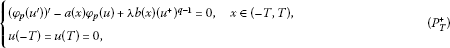where ${u}^{+}=max\left(u,0\right)$. It is easy to see that solutions of the problem (${P}_{T}^{+}$) are positive solutions of the problem (${P}_{T}$). Indeed, if $u\left(x\right)$ is a solution of (${P}_{T}^{+}$) and $u\left(x\right)$ has negative minimum at ${x}_{0}\in \left(-T,T\right)$, since for $p\ge 2$, ${\left({\phi }_{p}\left({u}^{\mathrm{\prime }}\right)\right)}^{\mathrm{\prime }}\left({x}_{0}\right)\ge 0$, by the equation ${\left({\phi }_{p}\left({u}^{\mathrm{\prime }}\right)\right)}^{\mathrm{\prime }}-a\left(x\right){\phi }_{p}\left(u\right)+\lambda b\left(x\right){\left({u}^{+}\right)}^{q-1}=0$, we reach a contradiction

$0={\left({\phi }_{p}\left({u}^{\mathrm{\prime }}\right)\right)}^{\mathrm{\prime }}\left({x}_{0}\right)+a\left({x}_{0}\right){\left(-u\left({x}_{0}\right)\right)}^{p-1}>0.$

Then $u\left(x\right)\ge 0$ and u is a solution of (${P}_{T}$). We use a variational treatment of the problem (${P}_{T}^{+}$), considering the functional ${J}_{T}:{X}_{T}\to \mathbb{R}$

${J}_{T}\left(u\right)={\int }_{-T}^{T}\left(\frac{1}{p}\left({|{u}^{\mathrm{\prime }}\left(x\right)|}^{p}+a\left(x\right){|u\left(x\right)|}^{p}\right)-\frac{\lambda }{q}b\left(x\right){\left({u}^{+}\left(x\right)\right)}^{q}\right)\phantom{\rule{0.2em}{0ex}}dx.$

Critical points of ${J}_{T}$ are weak solutions of (${P}_{T}^{+}$), i.e.,

${\int }_{-T}^{T}\left({\phi }_{p}\left({u}^{\mathrm{\prime }}\right){v}^{\mathrm{\prime }}+a\left(x\right){\phi }_{p}\left(u\right)v-\lambda b\left(x\right){\left({u}^{+}\right)}^{q-1}v\right)\phantom{\rule{0.2em}{0ex}}dx,\phantom{\rule{1em}{0ex}}\mathrm{\forall }v\in {W}_{0}^{1,p}\left(-T,T\right)$

and, by a standard way, they are solutions of (${P}_{T}^{+}$). We show that ${J}_{T}$ satisfies the assumptions of the mountain-pass theorem of Ambrosetti and Rabinowitz .

Theorem 6 (Mountain-pass theorem)

Let X be a Banach space with norm $\parallel \cdot \parallel$, $I\in {C}^{1}\left(X,\mathbf{R}\right)$, $I\left(0\right)=0$ and I satisfy the (PS) condition. Suppose that there exist $r>0$, $\alpha >0$ and $e\in X$ such that $\parallel e\parallel >r$

1. (i)

$I\left(x\right)\ge \alpha$ if $\parallel x\parallel =r$,

2. (ii)

$I\left(e\right)<0$. Let $c={inf}_{\gamma \in \mathrm{\Gamma }}\left\{{max}_{0\le t\le 1}I\left(\gamma \left(t\right)\right)\right\}\ge \alpha$, where

$\mathrm{\Gamma }=\left\{\gamma \in C\left(\left[0,1\right],X\right):\gamma \left(0\right)=0,\gamma \left(1\right)=e\right\}.$

Then c is a critical value of I, i.e., there exists ${x}_{0}$ such that $I\left({x}_{0}\right)=c$ and ${I}^{\mathrm{\prime }}\left({x}_{0}\right)=0$.

Next, denote by ${C}_{j}$ several positive constants.

Lemma 7 Let $2\le p, $\lambda >0$ and assumptions (H) hold. Then for every $T>0$, the problem (${P}_{T}$) has a positive solution ${u}_{T,\lambda }$. Moreover, there is a constant $K>0$, independent of T, such that

${\parallel {u}_{T,\lambda }\parallel }_{T}\le K.$
(8)

Proof Step 1. ${J}_{T}$ satisfies the (PS) condition.

Let ${\left({u}_{k}\right)}_{k}\subset {X}_{T}$ be a sequence, and suppose there exist ${C}_{1}$ and ${k}_{0}$ such that for $k\ge {k}_{0}$

$|{J}_{T}\left({u}_{k}\right)|=|{\int }_{-T}^{T}\left(\frac{1}{p}\left({|{u}_{k}^{\mathrm{\prime }}\left(x\right)|}^{p}+a\left(x\right){|{u}_{k}\left(x\right)|}^{p}\right)-\frac{\lambda }{q}b\left(x\right){\left({u}_{k}^{+}\left(x\right)\right)}^{q}\right)\phantom{\rule{0.2em}{0ex}}dx|\le \frac{{C}_{1}}{p},$
(9)

and

$|〈{J}_{T}\left({u}_{k}\right),{u}_{k}〉|=|{\int }_{-T}^{T}\left({|{u}_{k}^{\mathrm{\prime }}\left(x\right)|}^{p}+a\left(x\right){|{u}_{k}\left(x\right)|}^{p}-\lambda b\left(x\right){\left({u}_{k}^{+}\left(x\right)\right)}^{q}\right)\phantom{\rule{0.2em}{0ex}}dx|\le {\parallel {u}_{k}\parallel }_{T}.$
(10)

Let us denote $\stackrel{ˆ}{a}=min\left(1,a\right)$. From (9) and (10), it follows that

${C}_{1}\ge {\int }_{-T}^{T}\left(\left({|{u}_{k}^{\mathrm{\prime }}\left(x\right)|}^{p}+a\left(x\right){|{u}_{k}\left(x\right)|}^{p}\right)-\frac{\lambda p}{q}b\left(x\right){\left({u}_{k}^{+}\left(x\right)\right)}^{q}\right)\phantom{\rule{0.2em}{0ex}}dx\ge -{C}_{1}$

and

${\parallel {u}_{k}\parallel }_{T}\ge {\int }_{-T}^{T}\left(-{|{u}_{k}^{\mathrm{\prime }}\left(x\right)|}^{p}-a\left(x\right){|{u}_{k}\left(x\right)|}^{p}+\lambda b\left(x\right){\left({u}_{k}^{+}\left(x\right)\right)}^{q}\right)\phantom{\rule{0.2em}{0ex}}dx\ge -{\parallel {u}_{k}\parallel }_{T}$

Then

${C}_{1}+{\parallel {u}_{k}\parallel }_{T}\ge \lambda \frac{\left(q-p\right)b}{p}{\int }_{-T}^{T}{\left({u}_{k}^{+}\left(x\right)\right)}^{q}\phantom{\rule{0.2em}{0ex}}dx,$

and

$\begin{array}{rcl}\stackrel{ˆ}{a}{\parallel {u}_{k}\parallel }_{T}^{p}-{C}_{1}& \le & {\int }_{-T}^{T}\left({|{u}_{k}^{\mathrm{\prime }}\left(x\right)|}^{p}+a\left(x\right){|{u}_{k}\left(x\right)|}^{p}\right)\phantom{\rule{0.2em}{0ex}}dx-{C}_{1}\\ \le & \frac{\lambda p}{q}{\int }_{-T}^{T}b\left(x\right){\left({u}_{k}^{+}\left(x\right)\right)}^{q}\phantom{\rule{0.2em}{0ex}}dx\le \frac{\lambda pB}{q}{\int }_{-T}^{T}{\left({u}_{k}^{+}\left(x\right)\right)}^{q}\phantom{\rule{0.2em}{0ex}}dx.\end{array}$

We have

$\stackrel{ˆ}{a}{\parallel {u}_{k}\parallel }_{T}^{p}-{C}_{1}\le \frac{B}{q\left(q-p\right)b}\left({C}_{1}+{\parallel {u}_{k}\parallel }_{T}\right),$

which implies that the sequence ${\left({u}_{k}\right)}_{k}$ is bounded in ${X}_{T}$. By the compact embedding ${X}_{T}\subset C\left(\left[-T,T\right]\right)$, there exist $u\in {X}_{T}$ and the subsequence of ${\left({u}_{k}\right)}_{k}$, still denoted by ${\left({u}_{k}\right)}_{k}$, such that ${u}_{k}⇀u$ weakly in ${X}_{T}$ and ${u}_{k}\to u$ strongly in $C\left(\left[-T,T\right]\right)$. We will show that ${u}_{k}\to u$ strongly in ${X}_{T}$ using Lemma 2. By uniform convergence of ${u}_{k}$ to u in $C\left(\left[-T,T\right]\right)$, it follows that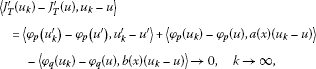and

$〈{\phi }_{p}\left({u}_{k}\right)-{\phi }_{p}\left(u\right),a\left(x\right)\left({u}_{k}-u\right)〉-〈{\phi }_{q}\left({u}_{k}\right)-{\phi }_{q}\left(u\right),b\left(x\right)\left({u}_{k}-u\right)〉\to 0,\phantom{\rule{1em}{0ex}}k\to \mathrm{\infty }.$

Then

$〈{\phi }_{p}\left({u}_{k}^{\mathrm{\prime }}\right)-{\phi }_{p}\left({u}^{\mathrm{\prime }}\right),{u}_{k}^{\mathrm{\prime }}-{u}^{\mathrm{\prime }}〉\to 0,\phantom{\rule{1em}{0ex}}k\to \mathrm{\infty },$

and by Lemma 2,

$〈{\phi }_{p}\left({u}_{k}^{\mathrm{\prime }}\right)-{\phi }_{p}\left({u}^{\mathrm{\prime }}\right),{u}_{k}^{\mathrm{\prime }}-{u}^{\mathrm{\prime }}〉\ge \left({|{u}_{k}^{\mathrm{\prime }}|}_{p}^{p-1}-{|{u}^{\mathrm{\prime }}|}_{p}^{p-1}\right)\left({|{u}_{k}^{\mathrm{\prime }}|}_{p}-{|{u}^{\mathrm{\prime }}|}_{p}\right)\ge 0,$

which implies that ${|{u}_{k}^{\mathrm{\prime }}|}_{p}\to {|{u}^{\mathrm{\prime }}|}_{p}$. Then ${\parallel {u}_{k}\parallel }_{T}\to {\parallel u\parallel }_{T}$ and by the uniform convexity of the space ${X}_{T}$, it follows that ${\parallel {u}_{k}-u\parallel }_{T}\to 0$, as $k\to \mathrm{\infty }$.

Step 2. Geometric conditions.

Obviously, ${J}_{T}\left(0\right)=0$. By assumption (H) it follows

$\begin{array}{rcl}{J}_{T}\left(u\right)& \ge & \frac{\stackrel{ˆ}{a}}{2p}{\parallel u\parallel }_{T}^{p}+{\int }_{-T}^{T}\left(\frac{a\left(x\right)}{2p}{|u\left(x\right)|}^{p}-\frac{\lambda p}{q}b\left(x\right){\left({u}^{+}\left(x\right)\right)}^{q}\right)\phantom{\rule{0.2em}{0ex}}dx\\ \ge & \frac{\stackrel{ˆ}{a}}{2p}{\parallel u\parallel }_{T}^{p}+{\int }_{-T}^{T}{|u\left(x\right)|}^{p}\left(\frac{a}{2p}-\frac{\lambda p}{q}b\left(x\right){|u\left(x\right)|}^{q-p}\right)\phantom{\rule{0.2em}{0ex}}dx>0\end{array}$

if ${\parallel u\parallel }_{T}=\rho :={\left(\frac{aq}{2\lambda {p}^{2}}\right)}^{1/\left(q-p\right)}>0$. Then ${J}_{T}\left(u\right)\ge \frac{\stackrel{ˆ}{a}{\rho }^{p}}{2p}>0$.

Let ${u}_{0}\left(x\right)\in {X}_{T}$ be such that ${u}_{0}\left(x\right)>0$ if $x\in \left(-T,T\right)$ and also ${u}_{0}\left(-T\right)={u}_{0}\left(T\right)=0$. Consider the function

Then

${J}_{T}\left({\stackrel{ˆ}{u}}_{0}\right)={\mu }^{p}{\int }_{-T}^{T}\frac{1}{p}\left({|{u}_{0}^{\mathrm{\prime }}\left(x\right)|}^{p}+a\left(x\right){|{u}_{0}\left(x\right)|}^{p}\right)\phantom{\rule{0.2em}{0ex}}dx-{\mu }^{q}{\int }_{-T}^{T}\frac{\lambda }{q}b\left(x\right){\left({u}_{0}\left(x\right)\right)}^{q}\phantom{\rule{0.2em}{0ex}}dx<0,$

for μ large enough.

By the mountain-pass theorem, there exists a solution ${u}_{T,\lambda }\in {X}_{T}$ such that

${c}_{T}={J}_{T}\left({u}_{T,\lambda }\right)=\underset{\gamma \in {\mathrm{\Gamma }}_{T}}{inf}\underset{t\in \left[0,1\right]}{max}{J}_{T}\left(\gamma \left(t\right)\right),\phantom{\rule{2em}{0ex}}{J}_{T}^{\mathrm{\prime }}\left({u}_{T,\lambda }\right)=0,$
(11)

where

${\mathrm{\Gamma }}_{T}=\left\{\gamma \left(t\right)\in C\left(\left[0,1\right],{X}_{T}\right):\gamma \left(0\right)=0,\gamma \left(1\right)={\stackrel{ˆ}{u}}_{0}\left(x\right)\right\}.$

Moreover, using the variational characterization (11), we have

${c}_{T}\ge \frac{\stackrel{ˆ}{a}{\rho }^{p}}{2p}>0.$

Therefore, ${u}_{T,\lambda }$ is a nontrivial and positive solution of (${P}_{T}$). By Theorem 4, $max\left\{{u}_{T,\lambda }:-T\le x\le T\right\}={u}_{T,\lambda }\left(0\right)$ and ${u}_{T,\lambda }^{\mathrm{\prime }}\left(x\right)<0$ for $x\in \left(0,T\right]$.

Step 3. Uniform estimates.

Let ${T}_{1}\ge T\ge 1$. By continuation with zero of a function $u\in {X}_{T}$ to $\left[-{T}_{1},{T}_{1}\right]$, we have ${X}_{T}\subset {X}_{{T}_{1}}$and ${\mathrm{\Gamma }}_{T}\subset {\mathrm{\Gamma }}_{{T}_{1}}$. Using the variational characterization (11), we infer that ${c}_{{T}_{1}}\le {c}_{T}\le {c}_{1}$ and then

${\int }_{-T}^{T}\left(\frac{1}{p}\left({|{u}_{T,\lambda }^{\mathrm{\prime }}\left(x\right)|}^{p}+a\left(x\right){u}_{T,\lambda }^{p}\left(x\right)\right)-\frac{\lambda }{q}b\left(x\right){u}_{T,\lambda }^{q}\left(x\right)\right)\phantom{\rule{0.2em}{0ex}}dx\le {c}_{1}.$
(12)

Multiplying the equation of (${P}_{T}$) by ${u}_{T}$ and integrating by parts, we have

${\int }_{-T}^{T}\left({|{u}_{T,\lambda }^{\mathrm{\prime }}|}^{p}+a\left(x\right){u}_{T,\lambda }^{p}\right)\phantom{\rule{0.2em}{0ex}}dx={\int }_{-T}^{T}\lambda b\left(x\right){u}_{T,\lambda }^{q}\phantom{\rule{0.2em}{0ex}}dx.$

Then by (12),

$\begin{array}{rcl}{c}_{1}& \ge & {\int }_{-T}^{T}\left(\frac{1}{p}\left({|{u}_{T,\lambda }^{\mathrm{\prime }}|}^{p}+a\left(x\right){u}_{T,\lambda }^{p}\right)-\frac{\lambda }{q}\lambda b\left(x\right){u}_{T,\lambda }^{q}\right)\phantom{\rule{0.2em}{0ex}}dx\\ \ge & \left(\frac{1}{p}-\frac{1}{q}\right){\int }_{-T}^{T}\left({|{u}_{T,\lambda }^{\mathrm{\prime }}|}^{p}+a\left(x\right){u}_{T,\lambda }^{p}\right)\phantom{\rule{0.2em}{0ex}}dx\ge \frac{\stackrel{ˆ}{a}\left(q-p\right)}{pq}{\parallel {u}_{T,\lambda }\parallel }_{T}^{p}.\end{array}$

We get (8) with $K=\frac{pq{c}_{1}}{\stackrel{ˆ}{a}\left(q-p\right)}$, which completes the proof. □

Proof of Theorem 1 Take ${T}_{n}\to \mathrm{\infty }$ and let ${u}_{n}$ be the solution of the problem (${P}_{{T}_{n}}$) given by Lemma 2. Consider the extension of ${u}_{n}$ to with zero outside $\left[-{T}_{n},{T}_{n}\right]$ and denote it by the same symbol.

Claim 1. The sequence of functions ${\left({u}_{n}\right)}_{n}$ is uniformly bounded and equicontinuous.

By (8) and the embedding of ${X}_{{T}_{n}}$ in $C\left(\left[-{T}_{n},{T}_{n}\right]\right)$, there is ${K}_{1}$ such that ${\parallel {u}_{n}\parallel }_{{L}^{\mathrm{\infty }}\left(\left[-{T}_{n},{T}_{n}\right]\right)}\le {K}_{1}$. Then by the equation of (${P}_{{T}_{n}}$), it follows that

${\parallel {\left({\phi }_{p}\left({u}_{n}^{\mathrm{\prime }}\right)\right)}^{\mathrm{\prime }}\parallel }_{{L}^{\mathrm{\infty }}\left(\left[-{T}_{n},{T}_{n}\right]\right)}\le {K}_{2}.$
(13)

By the mean value theorem for every natural n and every $t\in \mathbb{R}$, there exists ${\xi }_{n}\in \left[t-1,t\right]$ such that

${u}_{n}\left(t\right)-{u}_{n}\left(t-1\right)={u}_{n}^{\mathrm{\prime }}\left({\xi }_{k}\right).$

Then, as a consequence of (13), we obtain

$\begin{array}{rcl}|{\phi }_{p}\left({u}_{n}^{\mathrm{\prime }}\left(t\right)\right)|& =& |{\int }_{{\xi }_{k}}^{t}{\left({\phi }_{p}\left({u}_{n}^{\mathrm{\prime }}\left(s\right)\right)\right)}^{\mathrm{\prime }}\phantom{\rule{0.2em}{0ex}}ds+{\phi }_{p}\left({u}_{n}^{\mathrm{\prime }}\left({\xi }_{k}\right)\right)|\\ \le & {\int }_{t-1}^{t}|{\left({\phi }_{p}\left({u}_{n}^{\mathrm{\prime }}\left(s\right)\right)\right)}^{\mathrm{\prime }}|\phantom{\rule{0.2em}{0ex}}ds+{|{u}_{n}^{\mathrm{\prime }}\left({\xi }_{k}\right)|}^{p-1}\\ \le & {K}_{2}+{\left(|{u}_{n}\left(t\right)|+|{u}_{n}\left(t-1\right)|\right)}^{p-1}\\ \le & {K}_{2}+{\left(2{K}_{1}\right)}^{p-1}=:{K}_{3}^{\left(p-1\right)/p},\phantom{\rule{1em}{0ex}}\mathrm{\forall }t\in \mathbb{R},\end{array}$
(14)

from which it follows ${\parallel {u}_{n}^{\mathrm{\prime }}\parallel }_{{L}^{\mathrm{\infty }}\left(\left[-{T}_{n},{T}_{n}\right]\right)}\le {K}_{3}$ and the sequence of functions $\left({u}_{n}\right)$ is equicontinuous. Further, we claim that the sequence ${\left({u}_{n}^{\mathrm{\prime }}\right)}_{n}$ is also equicontinuous.

Claim 2. The sequence of functions ${\left({u}_{n}^{\mathrm{\prime }}\right)}_{n}$ is equicontinuous.

To prove this statement, we follow the method given by Tang and Xiao . For completeness, we present it in details.

Suppose that ${\left({u}_{n}^{\mathrm{\prime }}\right)}_{n}$ is not an equicontinuous sequence in ${C}_{\mathrm{loc}}\left(\mathbb{R}\right)$. Then there exist an ${\epsilon }_{0}$ and sequences $\left({t}_{k}^{1}\right)$ and $\left({t}_{k}^{2}\right)$ such that $0<{t}_{k}^{1}-{t}_{k}^{2}<\frac{1}{k}$ and

$|{u}_{n}^{\mathrm{\prime }}\left({t}_{k}^{1}\right)-{u}_{n}^{\mathrm{\prime }}\left({t}_{k}^{2}\right)|\ge {\epsilon }_{0}.$
(15)

By (14), there are numbers ${w}^{1}$ and ${w}^{2}$ and the subsequence $\left({u}_{{n}_{k}}^{\mathrm{\prime }}\right)$ such that ${u}_{{n}_{k}}^{\mathrm{\prime }}\left({t}_{k}^{1}\right)\to {w}^{1}$ and ${u}_{{n}_{k}}^{\mathrm{\prime }}\left({t}_{k}^{2}\right)\to {w}^{2}$ as $k\to \mathrm{\infty }$. By (15), $|{w}^{1}-{w}^{2}|\ge {\epsilon }_{0}$. On the other hand, by (13) we have

$|{\phi }_{p}\left({u}_{{n}_{k}}^{\mathrm{\prime }}\left({t}_{k}^{2}\right)\right)-{\phi }_{p}\left({u}_{{n}_{k}}^{\mathrm{\prime }}\left({t}_{k}^{1}\right)\right)|\le {\int }_{{t}_{k}^{1}}^{{t}_{k}^{2}}|{\phi }_{p}{\left({u}_{{n}_{k}}^{\mathrm{\prime }}\left(s\right)\right)}^{\mathrm{\prime }}|\phantom{\rule{0.2em}{0ex}}ds\le \frac{{K}_{2}}{k}.$

Then passing to a limit as $k\to \mathrm{\infty }$, we obtain ${\phi }_{p}\left({w}^{1}\right)={\phi }_{p}\left({w}^{2}\right)$. Hence, ${w}^{1}={w}^{2}$ which contradicts $|{w}^{1}-{w}^{2}|\ge {\epsilon }_{0}$. Thus, the sequence ${\left({u}_{n}^{\mathrm{\prime }}\right)}_{n}$ is equicontinuous.

Let $T>0$. By Claim 1 and Claim 2 and the Arzelà-Ascoli theorem, there is a subsequence of ${\left({u}_{n}\right)}_{n}$, still denoted by ${\left({u}_{n}\right)}_{n}$, and functions ${u}_{\lambda 1}$ and ${v}_{\lambda 1}$ of $C\left(\left[-T,T\right]\right)$ such that ${\parallel {u}_{n}-{u}_{\lambda 1}\parallel }_{C\left(\left[-T,T\right]\right)}\to 0$ and ${\parallel {u}_{n}^{\mathrm{\prime }}-{v}_{\lambda 1}\parallel }_{C\left(\left[-T,T\right]\right)}\to 0$. Trivially, it follows that ${u}_{\lambda 1}\in {C}^{1}\left(\left[-T,T\right]\right)$, ${u}_{\lambda 1}^{\mathrm{\prime }}={v}_{\lambda 1}$ and ${\parallel {u}_{n}-{v}_{\lambda 1}\parallel }_{{C}^{1}\left(\left[-T,T\right]\right)}\to 0$. Repeating this procedure as in , we obtain that there is a subsequence of ${\left({u}_{n}\right)}_{n}$, still denoted by ${\left({u}_{n}\right)}_{n}$, and ${u}_{\lambda }$ such that ${u}_{n}\to {u}_{\lambda }$ in ${C}_{\mathrm{loc}}^{1}\left(\mathbb{R}\right)$. The function ${u}_{\lambda }$ satisfies Eq. (1). Indeed, let $\left[{x}_{1},{x}_{2}\right]$ be an interval of and ${T}_{n}>0$ such that $\left[{x}_{1},{x}_{2}\right]\subset \left[-{T}_{n},{T}_{n}\right]$. By the above considerations, taking a limit as $n\to \mathrm{\infty }$ in the equation

${\left({u}_{n}^{\mathrm{\prime }}{|{u}_{n}^{\mathrm{\prime }}|}^{p-2}\right)}^{\mathrm{\prime }}-a\left(x\right){u}_{n}^{p-1}+\lambda b\left(x\right){u}_{n}^{q-1}=0,\phantom{\rule{1em}{0ex}}x\in \left[{x}_{1},{x}_{2}\right],$

equivalent to

$\begin{array}{rcl}{u}_{n}^{\mathrm{\prime }}{|{u}_{n}^{\mathrm{\prime }}|}^{p-2}\left(x\right)& =& {u}_{n}^{\mathrm{\prime }}{|{u}_{n}^{\mathrm{\prime }}|}^{p-2}\left({x}_{1}\right)+{\int }_{{x}_{1}}^{x}\left(a\left(t\right){u}_{n}^{p-1}\left(t\right)-\lambda b\left(t\right){u}_{n}^{q-1}\left(t\right)\right)\phantom{\rule{0.2em}{0ex}}dt\\ =& 0,\phantom{\rule{1em}{0ex}}x\in \left[{x}_{1},{x}_{2}\right],\end{array}$

we obtain

$\begin{array}{rcl}{u}_{\lambda }^{\mathrm{\prime }}{|{u}_{\lambda }^{\mathrm{\prime }}|}^{p-2}\left(x\right)& =& {u}_{\lambda }^{\mathrm{\prime }}{|{u}_{\lambda }^{\mathrm{\prime }}|}^{p-2}\left({x}_{1}\right)+{\int }_{{x}_{1}}^{x}\left(a\left(t\right){u}_{\lambda }^{p-1}\left(t\right)-\lambda b\left(t\right){u}_{\lambda }^{q-1}\left(t\right)\right)\phantom{\rule{0.2em}{0ex}}dt\\ =& 0,\phantom{\rule{1em}{0ex}}x\in \left[{x}_{1},{x}_{2}\right],\end{array}$

and hence

${\left({u}_{\lambda }^{\mathrm{\prime }}{|{u}_{\lambda }^{\mathrm{\prime }}|}^{p-2}\right)}^{\mathrm{\prime }}-a\left(x\right){u}_{\lambda }^{p-1}+\lambda b\left(x\right){u}_{\lambda }^{q-1}=0,\phantom{\rule{1em}{0ex}}x\in \left[{x}_{1},{x}_{2}\right].$

Since ${x}_{1}$ and ${x}_{2}$ are arbitrary, ${u}_{\lambda }$ is a solution of (1). Moreover, we have

${\int }_{-\mathrm{\infty }}^{\mathrm{\infty }}\left({|{u}_{\lambda }^{\mathrm{\prime }}\left(x\right)|}^{p}+a\left(x\right){|{u}_{\lambda }\left(x\right)|}^{p}\right)\phantom{\rule{0.2em}{0ex}}dx<\mathrm{\infty }.$
(16)

It remains to show that ${u}_{\lambda }$ is nonzero and ${u}_{\lambda }\left(±\mathrm{\infty }\right)=0$ and ${u}_{\lambda }^{\mathrm{\prime }}\left(±\mathrm{\infty }\right)=0$.

By Theorem 4, ${u}_{n}$ is an even function and attains its maximum at 0. Then by Eq. (1),

${u}_{n}^{p-1}\left(0\right)\left(-a\left(0\right)+\lambda b\left(0\right){u}_{n}^{q-p}\left(0\right)\right)\ge 0.$

By assumption (H)

${u}_{n}\left(0\right)\ge {\left(\frac{a\left(0\right)}{\lambda b\left(0\right)}\right)}^{1/\left(q-p\right)}\ge {\left(\frac{a}{\lambda B}\right)}^{1/\left(q-p\right)}={C}_{3}>0,$

independently of n. Hence, passing to a limit as $n\to \mathrm{\infty }$, we obtain

${u}_{\lambda }\left(0\right)\ge {\left(\frac{a}{\lambda B}\right)}^{1/\left(q-p\right)}>0.$

Note, that this implies $max\left\{{u}_{\lambda }\left(x\right):x\in R\right\}={u}_{\lambda }\left(0\right)\to +\mathrm{\infty }$ as $\lambda \to 0$.

From (16) and Proposition 5, it follows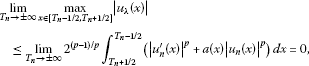(17)

so ${u}_{\lambda }\left(±\mathrm{\infty }\right)=0$.

Now, we will show that ${u}_{\lambda }^{\mathrm{\prime }}\left(\mathrm{\infty }\right)=0$. The arguments for ${u}_{\lambda }^{\mathrm{\prime }}\left(-\mathrm{\infty }\right)=0$ are similar.

If ${u}_{\lambda }^{\mathrm{\prime }}\left(\mathrm{\infty }\right)\ne 0$, there exist ${\epsilon }_{1}>0$ and a monotone increasing sequence ${x}_{k}\to \mathrm{\infty }$ such that $|{u}_{\lambda }^{\mathrm{\prime }}\left({x}_{k}\right)|\ge {\left(2{\epsilon }_{1}\right)}^{1/\left(p-1\right)}$. Then for $x\in \left[{x}_{k},{x}_{k}+\frac{{\epsilon }_{1}}{{K}_{2}}\right]$,

$\begin{array}{rcl}{|{u}_{\lambda }^{\mathrm{\prime }}\left(x\right)|}^{p-1}& =& |{\phi }_{p}\left({u}_{\lambda }^{\mathrm{\prime }}\left({x}_{k}\right)\right)+{\int }_{{x}_{k}}^{x}{\phi }_{p}{\left({u}_{\lambda }^{\mathrm{\prime }}\left(t\right)\right)}^{\mathrm{\prime }}\phantom{\rule{0.2em}{0ex}}dt|\\ \ge & {|{u}_{\lambda }^{\mathrm{\prime }}\left({x}_{k}\right)|}^{p-1}-{\int }_{{x}_{k}}^{{x}_{k}+\frac{{\epsilon }_{1}}{{K}_{2}}}|{\phi }_{p}{\left({u}_{\lambda }^{\mathrm{\prime }}\left(t\right)\right)}^{\mathrm{\prime }}|\phantom{\rule{0.2em}{0ex}}dt\\ \ge & 2{\epsilon }_{1}-\frac{{\epsilon }_{1}}{{K}_{2}}\cdot {K}_{2}={\epsilon }_{1},\end{array}$

Moreover, u is an even function that attains its only maximum at 0, since the same holds for the functions ${u}_{n}$. Arguing as in the proof of Theorem 4, we easily obtain that ${u}^{\mathrm{\prime }}\left(x\right)<0$ if $x>0$. □

Remark 2 A simplified method can be applied to the equations

${u}^{\mathrm{\prime }\mathrm{\prime }}-a\left(x\right)u{|u|}^{p-2}+\lambda b\left(x\right)u{|u|}^{q-2}=0,\phantom{\rule{1em}{0ex}}x\in \mathbb{R},$

under assumptions (H) and $2\le p, $\lambda >0$. Namely, first one looks for the even positive solutions ${u}_{T,\lambda }$ of the problem

$\left\{\begin{array}{c}{u}^{\mathrm{\prime }\mathrm{\prime }}-a\left(x\right){\phi }_{p}\left(u\right)+\lambda b\left(x\right){\phi }_{q}\left(u\right)=0,\phantom{\rule{1em}{0ex}}x\in \left(-T,T\right),\hfill \\ u\left(-T\right)=u\left(T\right)=0,\hfill \end{array}$

considering the functional ${I}_{T}:{H}_{0}^{1}\left(-T,T\right)\to \mathbb{R}$

${I}_{T}\left(u\right)={\int }_{-T}^{T}\left(\frac{1}{2}{u}^{\mathrm{\prime }}{\left(x\right)}^{2}+\frac{1}{p}a\left(x\right){|u\left(x\right)|}^{p}-\frac{\lambda }{q}b\left(x\right){\left({u}^{+}\left(x\right)\right)}^{q}\right)\phantom{\rule{0.2em}{0ex}}dx,$

where ${H}_{0}^{1}\left(-T,T\right)$ is the Sobolev space of square integrable functions such that

${\parallel u\parallel }^{2}={\int }_{-T}^{T}{u}^{\mathrm{\prime }}{\left(x\right)}^{2}\phantom{\rule{0.2em}{0ex}}dx<\mathrm{\infty }.$

Since ${H}_{0}^{1}\left(-T,T\right)$ is a Hilbert space, compactly embedded in $C\left(\left[-T,T\right]\right)$ the proof of the (PS)-condition is easier. Similar considerations are made in  and . Then, the even homoclinic solution ${u}_{\lambda }$ is obtained as a ${C}_{\mathrm{loc}}^{1}$ limit of the sequence ${u}_{T,\lambda }$. Note that in this case, the even homoclinic solution ${u}_{\lambda }$ of Eq. (3) satisfies

$max\left\{{u}_{\lambda }\left(x\right):x\in \mathbb{R}\right\}={u}_{\lambda }\left(0\right)\ge {\left(\frac{a\left(0\right)}{\lambda b\left(0\right)}\right)}^{1/\left(q-p\right)},$

and again ${u}_{\lambda }\left(0\right)\to +\mathrm{\infty }$ as $\lambda \to 0$. If a and b are constants, Eq. (3) is a conservative system and one can plot the phase curves ${\left(\frac{v}{2}\right)}^{2}-a\frac{{|u|}^{p}}{p}+\lambda b\frac{{|u|}^{q}}{q}=C$ in the phase plane $\left(u,v\right)=\left(u,{u}^{\mathrm{\prime }}\right)$. Consider the equation ${u}^{\mathrm{\prime }\mathrm{\prime }}-{u}^{3}+\lambda {u}^{5}=0$. The phase portrait in a $\left(u,v\right)$ plane, for $\lambda =0.5$ in the rectangle $\left\{\left(u,v\right):-2\le u\le 2,-1.25\le v\le 1.25\right\}$, is plotted on Figure 2.

## References

1. Korman P, Lazer A: Homoclinic orbits for a class of symmetric hamiltonian systems. Electron. J. Differ. Equ. 1994., 1994: Article ID 1

2. Grossinho MR, Sanchez L: A note on periodic solutions of some nonautonomous differential equations. Bull. Aust. Math. Soc. 1986, 34: 253-265. 10.1017/S000497270001011X

3. Grossinho MR, Minhos F, Tersian S: Positive homoclinic solutions for a class of second order differential equations. J. Math. Anal. Appl. 1999, 240: 163-173. 10.1006/jmaa.1999.6606

4. Austin G: Biomathematical model of aneurysm of the circle of Willis I: the Duffing equation and some approximate solutions. Math. Biosci. 1971, 11: 163-172. 10.1016/0025-5564(71)90015-0

5. Cronin J: Biomathematical model of aneurysm of the circle of Willis: a quantitative analysis of the differential equation of Austin. Math. Biosci. 1973, 16: 209-225. 10.1016/0025-5564(73)90031-X

6. Nieto JJ, Torres A: A nonlinear biomathematical model for the study of intracranial aneurysms. J. Neurol. Sci. 2000, 177: 18-23. 10.1016/S0022-510X(00)00315-4

7. Tang XH, Li X: Homoclinic solutions for ordinary p -Laplacian systems with a coercive potential. Nonlinear Anal. 2009, 71: 1124-1132. 10.1016/j.na.2008.11.027

8. Xu B, Tang C-L: Some existence results on periodic solutions of ordinary p -Laplacian systems. J. Math. Anal. Appl. 2007, 333: 1228-1236. 10.1016/j.jmaa.2006.11.051

9. Korman P, Ouyang T: Exact multiplicity results for two classes of boundary value problems. Differ. Integral Equ. 1993, 6(6):1507-1517.

10. Gidas B, Ni WM, Nirenberg L: Symmetry and related properties via the maximum principle. Commun. Math. Phys. 1979, 68: 209-243. 10.1007/BF01221125

11. Reihel W, Walter W: Radial solutions of equations and inequalities involving the p -Laplacian. J. Inequal. Appl. 1997, 1: 47-71.

12. Cabada A, Cid JA, Pouso RL: Positive solutions for a class of singular differential equations arising in diffusion processes. Dyn. Contin. Discrete Impuls. Syst. Ser. A, Math. Anal. 2005, 12: 329-342.

13. Rabinowitz P: Homoclinic orbits for a class of Hamiltonian systems. Proc. R. Soc. Edinb. A 1990, 114: 33-38. 10.1017/S0308210500024240

14. Aizicovici S, Papageorgiou NS, Staicu V: Periodic solutions of nonlinear evolution inclusions in Banach spaces. J. Nonlinear Convex Anal. 2011, 7(2):163-177.

15. Del Pino M, Drabek P, Manásevich R: The Fredholm alternative at the first eigenvalue for the one-dimensional p -Laplacian. J. Differ. Equ. 1999, 151: 386-419. 10.1006/jdeq.1998.3506

16. Ambrosetti A, Rabinowitz P: Dual variational methods in critical point theory and applications. J. Funct. Anal. 1973, 14: 349-381. 10.1016/0022-1236(73)90051-7

## Acknowledgements

Dedicated to Professor Jean Mawhin on the occasion of his 70th anniversary.

The author thanks Prof. Alberto Cabada and Prof. Luis Sanchez for helpful remarks concerning Theorem 4. The author would like to thank the Department of Mathematics and Theoretical Informatics at the Technical University of Kosice, Slovakia, where the paper was prepared during his visit on the SAIA Fellowship programme. The author is thankful to the editor and anonymous referee for their comments and suggestions on the article.

## Author information

Authors

### Corresponding author

Correspondence to Stepan Tersian.

### Competing interests

The author declares that he has no competing interests.

## Authors’ original submitted files for images

Below are the links to the authors’ original submitted files for images.

## Rights and permissions

Reprints and Permissions

Tersian, S. On symmetric positive homoclinic solutions of semilinear p-Laplacian differential equations. Bound Value Probl 2012, 121 (2012). https://doi.org/10.1186/1687-2770-2012-121

• Accepted:

• Published:

• DOI: https://doi.org/10.1186/1687-2770-2012-121

### Keywords

• p-Laplacian ODEs
• homoclinic solution
• weak solution
• Palais-Smale condition
• mountain-pass theorem